# New demand ppt

7 Oct 2010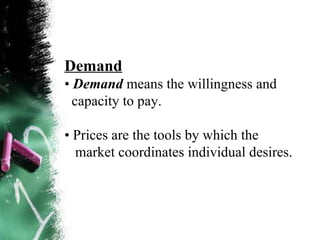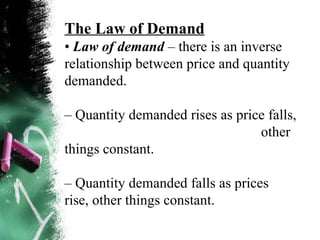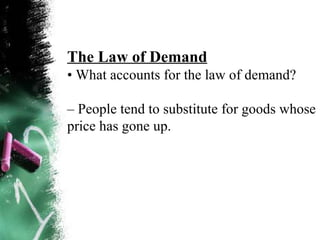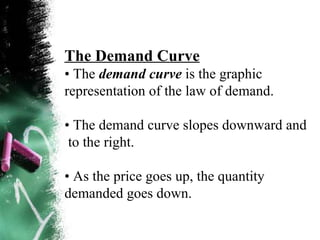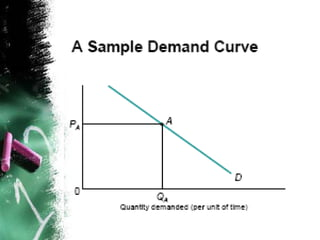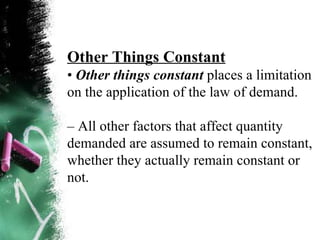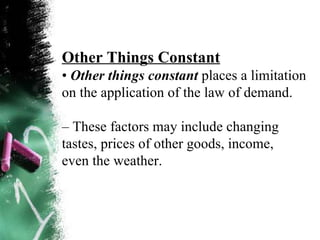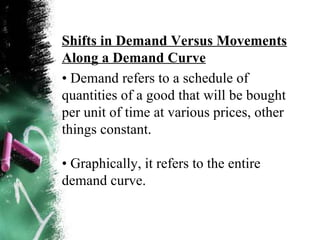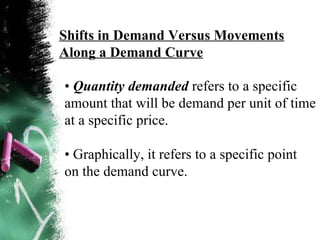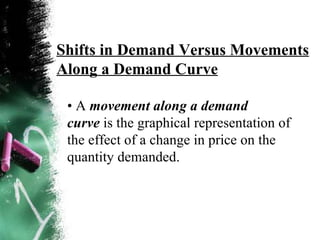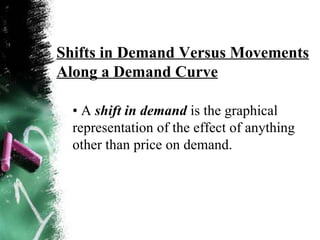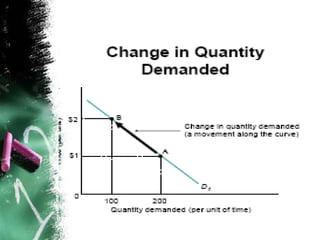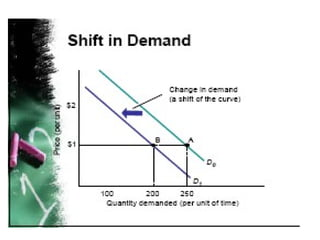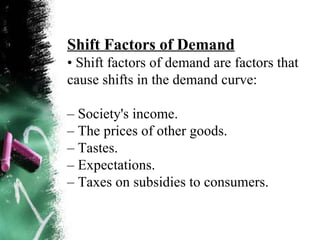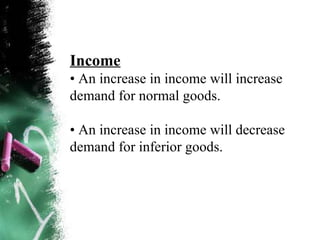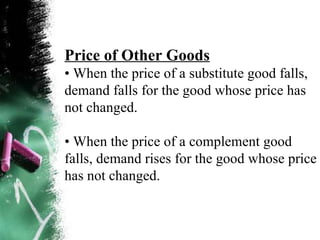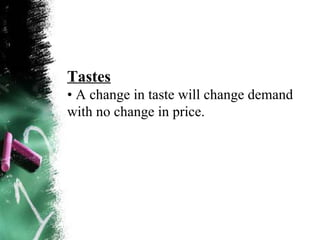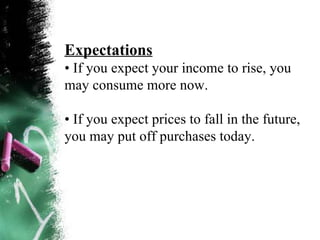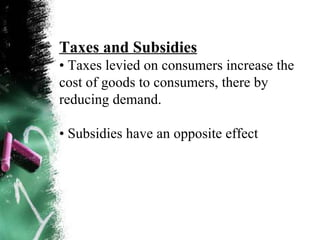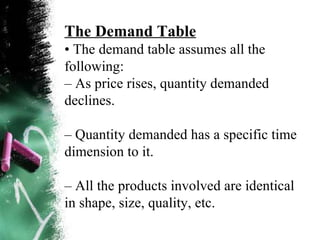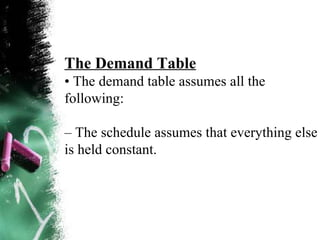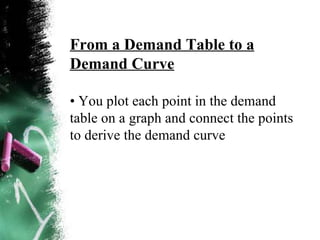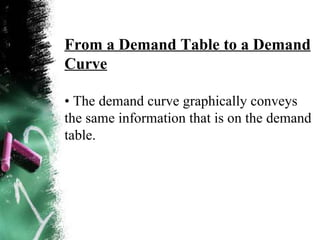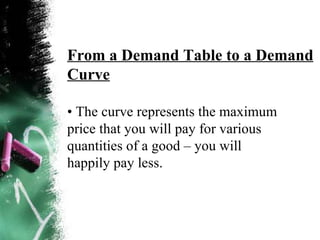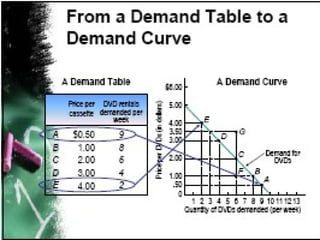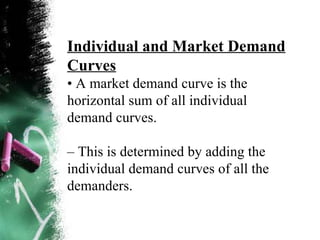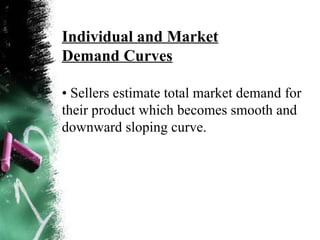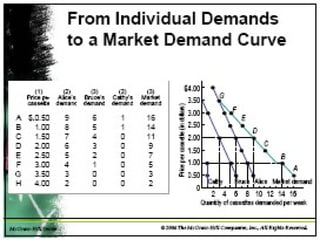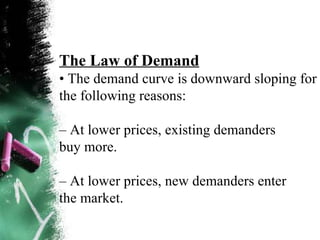1 sur 31

### New demand ppt

• 2. Laugher Curve Q. What do you get when you cross the Godfather with an economist? A. An offer you can't understand
• 3. Demand • Demand means the willingness and capacity to pay. • Prices are the tools by which the market coordinates individual desires.
• 4. The Law of Demand • Law of demand – there is an inverse relationship between price and quantity demanded. – Quantity demanded rises as price falls, other things constant. – Quantity demanded falls as prices rise, other things constant.
• 5. The Law of Demand • What accounts for the law of demand? – People tend to substitute for goods whose price has gone up.
• 6. The Demand Curve • The demand curve is the graphic representation of the law of demand. • The demand curve slopes downward and to the right. • As the price goes up, the quantity demanded goes down.
• 7.
• 8. Other Things Constant • Other things constant places a limitation on the application of the law of demand. – All other factors that affect quantity demanded are assumed to remain constant, whether they actually remain constant or not.
• 9. Other Things Constant • Other things constant places a limitation on the application of the law of demand. – These factors may include changing tastes, prices of other goods, income, even the weather.
• 10. • Demand refers to a schedule of quantities of a good that will be bought per unit of time at various prices, other things constant. • Graphically, it refers to the entire demand curve. Shifts in Demand Versus Movements Along a Demand Curve
• 11. • Quantity demanded refers to a specific amount that will be demand per unit of time at a specific price. • Graphically, it refers to a specific point on the demand curve. Shifts in Demand Versus Movements Along a Demand Curve
• 12. Shifts in Demand Versus Movements Along a Demand Curve • A movement along a demand curve is the graphical representation of the effect of a change in price on the quantity demanded.
• 13. Shifts in Demand Versus Movements Along a Demand Curve • A shift in demand is the graphical representation of the effect of anything other than price on demand.
• 14.
• 15.
• 16. Shift Factors of Demand • Shift factors of demand are factors that cause shifts in the demand curve: – Society's income. – The prices of other goods. – Tastes. – Expectations. – Taxes on subsidies to consumers.
• 17. Income • An increase in income will increase demand for normal goods. • An increase in income will decrease demand for inferior goods.
• 18. Price of Other Goods • When the price of a substitute good falls, demand falls for the good whose price has not changed. • When the price of a complement good falls, demand rises for the good whose price has not changed.
• 19. Tastes • A change in taste will change demand with no change in price.
• 20. Expectations • If you expect your income to rise, you may consume more now. • If you expect prices to fall in the future, you may put off purchases today.
• 21. Taxes and Subsidies • Taxes levied on consumers increase the cost of goods to consumers, there by reducing demand. • Subsidies have an opposite effect
• 22. The Demand Table • The demand table assumes all the following: – As price rises, quantity demanded declines. – Quantity demanded has a specific time dimension to it. – All the products involved are identical in shape, size, quality, etc.
• 23. The Demand Table • The demand table assumes all the following: – The schedule assumes that everything else is held constant.
• 24. From a Demand Table to a Demand Curve • You plot each point in the demand table on a graph and connect the points to derive the demand curve
• 25. From a Demand Table to a Demand Curve • The demand curve graphically conveys the same information that is on the demand table.
• 26. From a Demand Table to a Demand Curve • The curve represents the maximum price that you will pay for various quantities of a good – you will happily pay less.
• 27.
• 28. Individual and Market Demand Curves • A market demand curve is the horizontal sum of all individual demand curves. – This is determined by adding the individual demand curves of all the demanders.
• 29. Individual and Market Demand Curves • Sellers estimate total market demand for their product which becomes smooth and downward sloping curve.
• 30.
• 31. The Law of Demand • The demand curve is downward sloping for the following reasons: – At lower prices, existing demanders buy more. – At lower prices, new demanders enter the market.